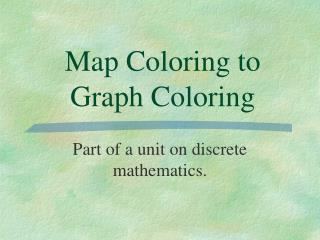DownloadDownload PresentationMap Coloring to Graph Coloring

Map Coloring to Graph Coloring

Download PresentationMap Coloring to Graph Coloring

- - - - - - - - - - - - - - - - - - - - - - - - - - - E N D - - - - - - - - - - - - - - - - - - - - - - - - - - -
Presentation Transcript

1. Map Coloring to Graph Coloring Part of a unit on discrete mathematics.

2. Discrete math: What is it? • It is mathematics which studies phenomena which are not continuous, but happens in small, or discrete, chunks. • Some areas include graph theory (networks), counting techniques, coloring theory, game theory, and more. • It is the mathematics of computers. • It is finite, rather than infinite. It handles distinct chunks of information. You might talk about the schedule of flights leaving Chicago, but not the acceleration of one of those planes.

3. A map is a two-dimensional drawing with regions to be colored. A graph is a mathematical object made of dots connected by lines. Maps versus Graphs

4. Graphs in Computer Science • The term graph is used in a different sense in mathematics to mean a chart displaying numerical data, such as a bar graph, but the graphs that computer scientists use are not related to these. • In computer science, graphs are drawn using circles or large dots, technically called "nodes," to denote objects. • Lines between nodes indicate some sort of relationship between the objects.

5. We can represent the map of South America using a graph: • Each circle represents a country. • Countries which share a common border on the map are connected by lines called edges on the graph.

6. We have been studying map coloring in class. Recall the rules of map coloring: • Regions (or countries) which share a border must be colored different colors. • Regions which touch at only one point at a time may be colored the same color. • You must use the least amount of colors possible.

7. We can study graph coloring as well: • Dots (or nodes) represent the regions, and a line between two nodes indicates that those two regions share a border. • On the graph, the coloring rule is that no connected nodes should be colored the same color. • Unlike a map, there is no limit to the number of colors that a general graph may require. • The "graph coloring problem" is to find the minimum number of colors that are needed for a particular graph. • This minimum number of colors needed to color a graph is called the chromatic number of the graph.

8. Try coloring our Lands map using a graph: The graph can be colored with two colors, just like the map! The chromatic number of this graph is 2. Now try coloring the South America map using a graph!

9. Here is another graph: • A line between two subjects indicates that at least one student is taking both subjects, and so they should not be scheduled for the same period. Using this representation, the problem of finding a workable timetable using the minimum number of periods is equivalent to the coloring problem, where the different colors correspond to different periods. What is the chromatic number of this graph?

10. What are some other ways graphs and graph coloring are used to organize information? • Round-Robin Tournaments • Precedence Graphs (graphs which determine the order in which to complete tasks) • Logic Puzzles • Television and Radio Frequencies • See this example on the Web • Fish and Fish Tanks: also on the Web • Many more applications can be found by following this link!

11. Now it’s time for a quiz! • Click below for a fun and invigorating quiz show game! Quiz Show